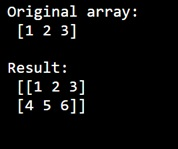# Stack summing vectors to numpy 3d array

Learn, how to stack vectors to numpy 3d array?
Submitted by Pranit Sharma, on December 30, 2022

NumPy is an abbreviated form of Numerical Python. It is used for different types of scientific operations in python. Numpy is a vast library in python which is used for almost every kind of scientific or mathematical operation. It is itself an array which is a collection of various methods and functions for processing the arrays.

## Stack vectors to numpy 3d array

Suppose we are given two NumPy arrays and we need to stack them together in such a way that it becomes a two-dimensional array.

For this purpose, we will use the numpy.stack() method. This method is used for joining multiple NumPy arrays. Unlike, concatenate(), it joins arrays along a new axis. It returns a NumPy array.

Let us understand with the help of an example,

## Python code for 'stack summing vectors to numpy 3d array'

```# Import numpy
import numpy as np

# Import pandas
import pandas as pd

# Creating a numpy array
arr = np.array([1, 2, 3])

# Display original array
print("Original array:\n",arr,"\n")

# Creating another numpy array
arr2 = np.array([4, 5, 6])

# Stacking the two arrays
res = np.stack((arr, arr2),axis=0)

# Display result
print("Result:\n",res,"\n")
```

Output: